Get instant live expert help with Excel or Google Sheets“My Excelchat expert helped me in less than 20 minutes, saving me what would have been 5 hours of work!”

#### Post your problem and you’ll get expert help in seconds.

Your message must be at least 40 characters
Our professional experts are available now. Your privacy is guaranteed.

# How to Show Formulas Instead of Values or Results

When we type a formula in Excel and press the enter key, it will return with the calculated result, while the formula may be seen in the formula bar. However, it is possible to display formulas rather than the calculated result. In this tutorial, we will learn how to show formula in a single cell, selected cells, or print formulas.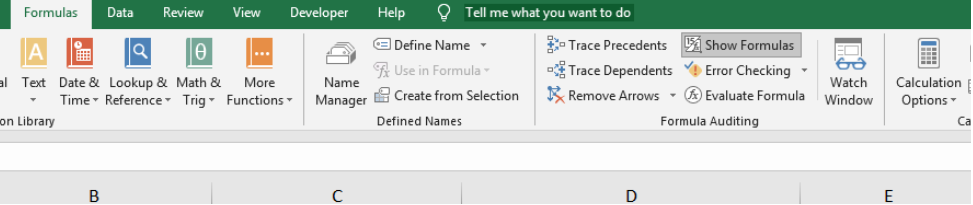Figure 1 – How to show all formulas instead of the result

## Show Formula in Excel rather than values

• We will prepare a data table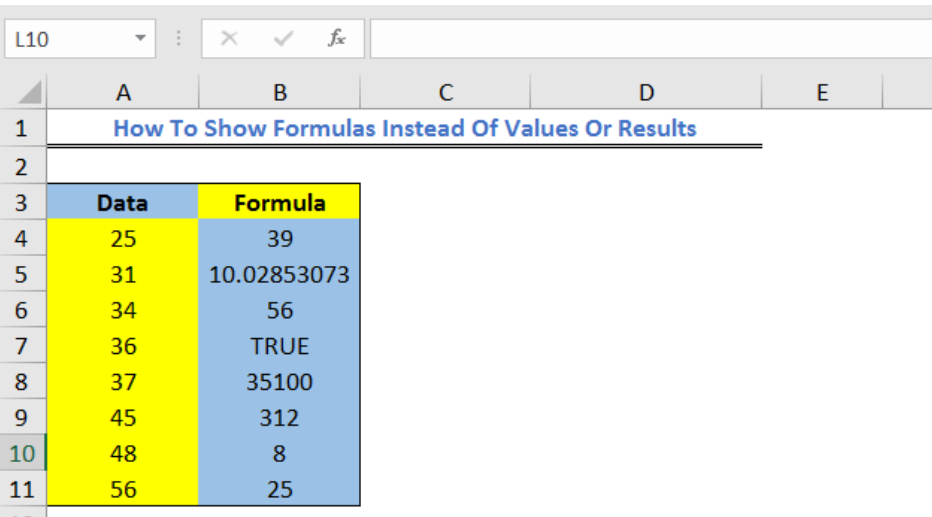Figure 2 – Show formula in excel

• We will click on the Formula Tab and select Show Formulas from the Formula Auditing group.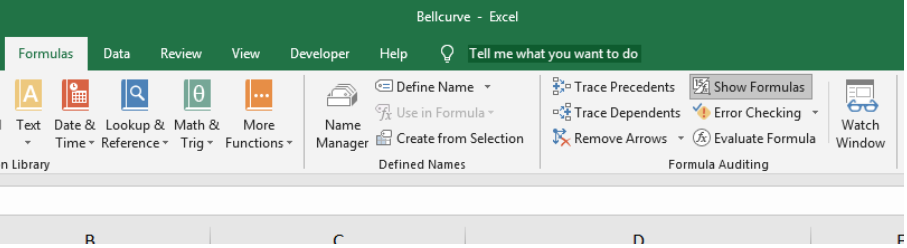Figure 3 – How to display formulas in excel

• Excel Instantly returns with the formulas in our cells rather than their results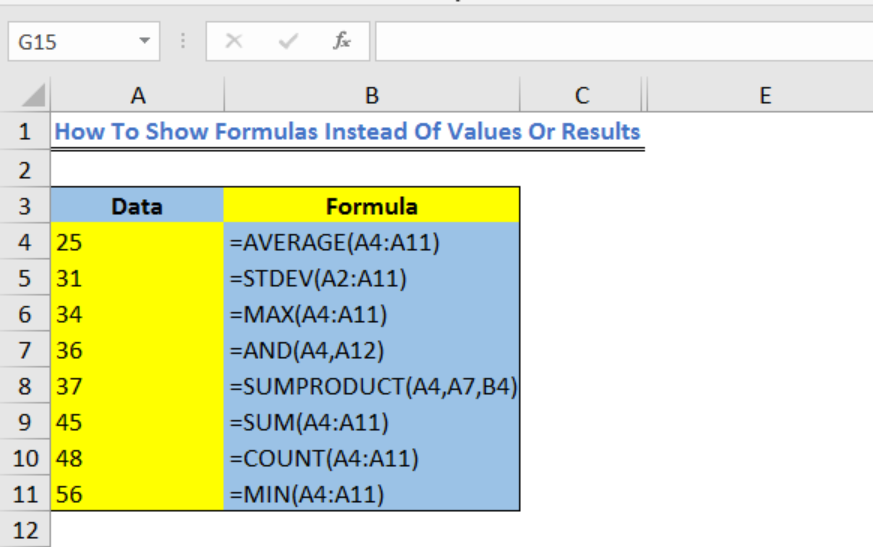Figure 4 – Excel Showing formula instead of value

• Alternatively, we can use the show formulas excel shortcut. We will click on the cell whose formula we want to show and press the short cut key (Ctrl +)

## Show Formula in Worksheets in Excel

If we have lots of worksheets and wish to show formula in all cells in that worksheet, here’s what we will do;

• We will go to the File Tab and select Options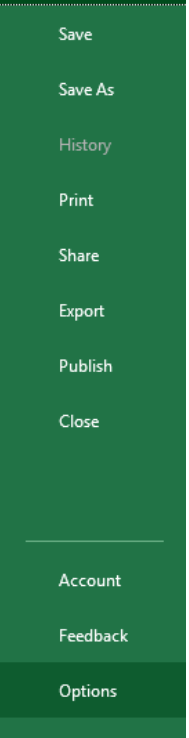Figure 5 – How do you display formulas in excel

• In the left pane, we will select Advanced and scroll down to Display Options for this worksheet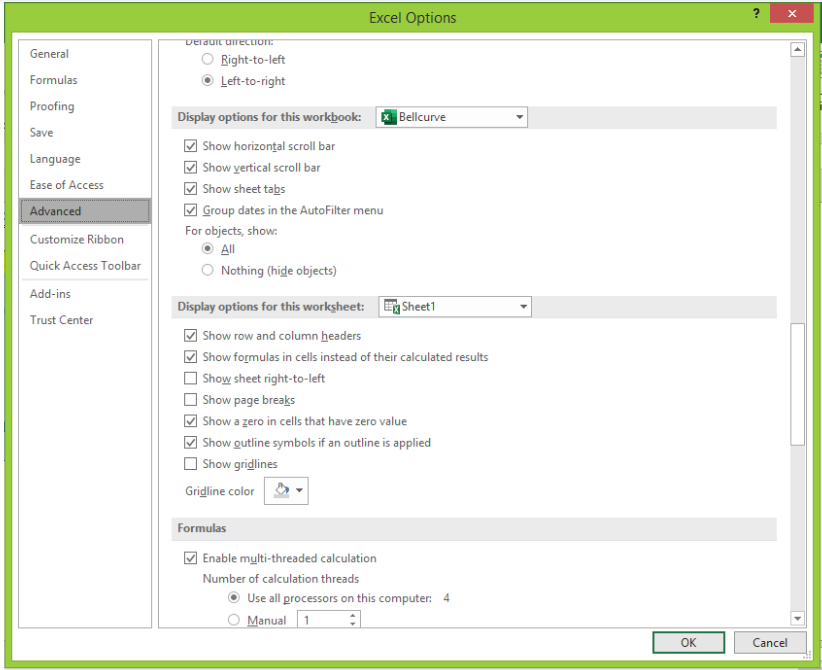Figure 6 – Show cells in excel

• In the section menu, we will select the worksheet we want to Show formulas in cells instead of their calculated results.

• We will click OK.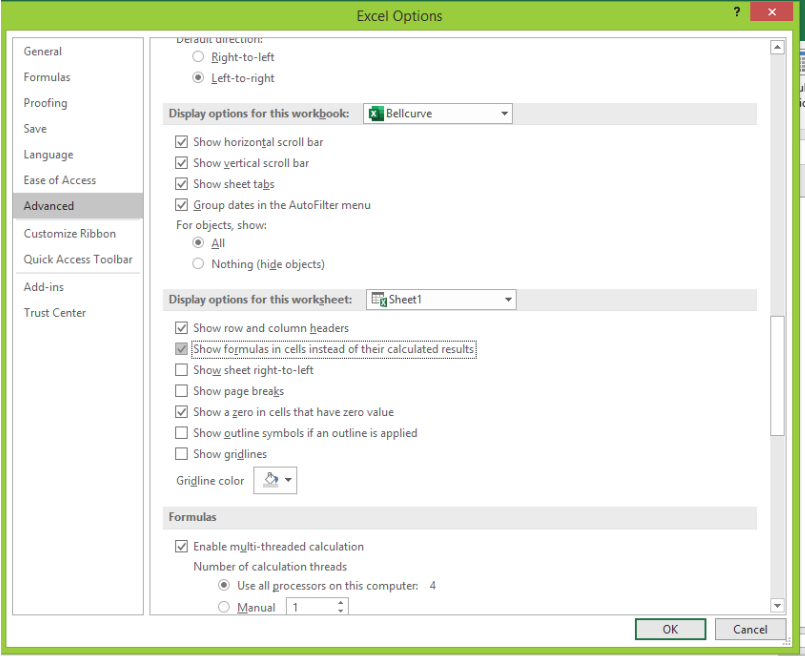Figure 7 – Excel cells show formula instead of the result

## Show Formula in Selected Cells

• We will go to Home, choose to Find & Select and pick Replace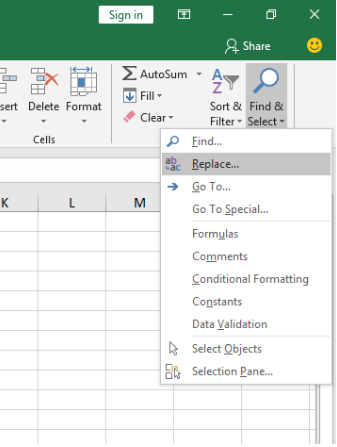Figure 8 – Excel showing formula instead of value

• In the Find and Replace dialog box, we will need the symbol “=” in Find what field and Replace with field.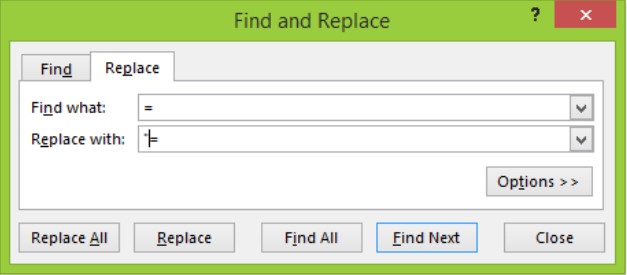Figure 9 – How to show all formulas in excel

• We will click on Replace All.
• Now, we can see all the formulas in the selected cells.

Note – If we click a cell with a formula and the formula does not show up, then it is possible that the formula is hidden and the worksheet is protected.

## Instant Connection to an Excel Expert

Most of the time, the problem you will need to solve will be more complex than a simple application of a formula or function. If you want to save hours of research and frustration, try our live Excelchat service! Our Excel Experts are available 24/7 to answer any Excel question you may have. We guarantee a connection within 30 seconds and a customized solution within 20 minutes.